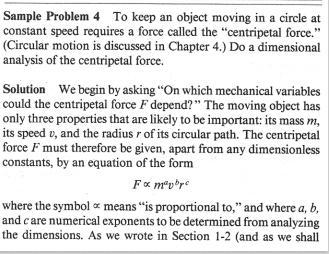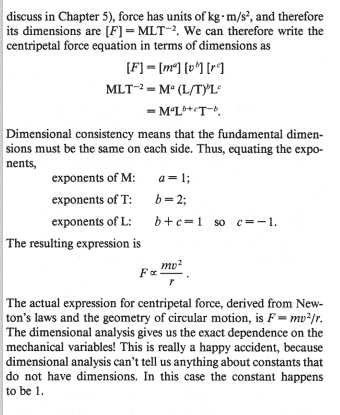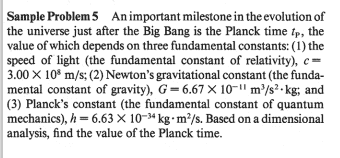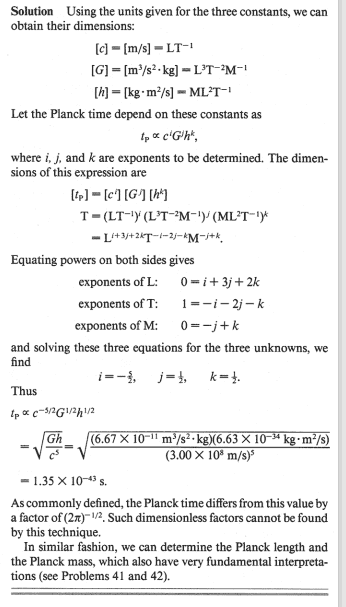# Dimensional analysis assumption

walking
Problem:Source: Halliday et al Physics 4e, p9, sample problem 4.

Why is it valid to assume that $$F\alpha v^b$$ as the author does here, and not some more complex equation e.g. $$F=av^{e_1}+bm^{e_2}+cr^{e_3}$$, or $$F=av^{e_1}+bv^{e_2}+\cdots$$ (or some other equation)?

A very similar question I had is from same source, page 10, sample problem 5:Again, why can authors assume that the variables appear as a product without there being any summing involved?

I believe, for your examples, this follows from the Buckingham Pi theorem. In both of your examples, you have 4 variables ##q_i## and 3 independent physical dimensions (##M, L, T##). You can hence form ##4-3=1## dimensionless group ##\Pi = {q_1}^{\alpha} {q_2}^{\beta}{q_3}^{\gamma}{q_4}^{\delta}## for some ##\alpha, \beta, \gamma, \delta## to be determined, and by the Pi theorem the relationship must take the form$$f(\Pi) = 0$$for some function ##f##. Inverting this relationship,$$\Pi = {q_1}^{\alpha} {q_2}^{\beta}{q_3}^{\gamma}{q_4}^{\delta} = f^{-1}(0)= K$$where ##K = f^{-1}(0)## is a constant. So the relationship between the ##q_i## must take the form$$q_1 = K^{\frac{1}{\alpha}} {q_2}^{-\frac{\beta}{\alpha}}{q_3}^{-\frac{\gamma}{\alpha}}{q_4}^{-\frac{\delta}{\alpha}} \equiv C {q_2}^{a} {q_3}^{b} {q_4}^{c}$$for some new constants ##C##, ##a##, ##b##, ##c##.

Last edited by a moderator:
•sysprog
2022 Award
LaTeX point - \propto gets you the ##\propto## symbol, which LaTeX then spaces similar to an = sign rather than a symbol. So ##F\propto v^b##, rather than ##F\alpha v^b##.
$$F=av^{e_1}+bm^{e_2}+cr^{e_3}$$
If this were the case, what would ##F## be if ##v=0## but ##m\neq 0## and ##r\neq 0##? Does that make sense?
$$F=av^{e_1}+bv^{e_2}+\cdots$$
Here you have no dependence on mass at all. Is it your experience that swinging a one kilogram mass on a string is just as easy as swinging a one ton mass? If not, is this equation plausible?

That said, you are correct to be suspicious. For example, if you consider kinetic energy, you might apply this analysis and conclude that ##E\propto mv^2##. With a bit of experiment you could find that the constant of proportionality is 1/2, so ##E=\frac 12mv^2##. Great! Unfortunately, Einstein showed that a more precise formula for kinetic energy is ##E=(\gamma-1)mc^2##, where ##\gamma=\frac{1}{\sqrt{1-v^2/c^2}}##. If you do a Taylor series expansion of ##\gamma## you'll find that $$E=(\gamma-1)mc^2\approx \frac 12mv^2+\frac 38m\frac{v^4}{c^2}+\ldots$$At every day velocities that second term is negligible and it's completely understandable that we were unaware of it until we started studying things that move at or near lightspeed. But there's no way to get to it (or to the full formula) by dimensional analysis.

So the moral of the story is that dimensional analysis will always give you a plausible answer. In Newtonian physics, it will often give you the correct answer. It's a useful sanity check on any formula you get from any process (if the dimensions don't match, it's wrong). But it isn't in any sense foolproof.

•sysprog and etotheipi
walking
I believe, for your examples, this follows from the Buckingham Pi theorem. In both of your examples, you have 4 variables ##q_i## and 3 independent physical dimensions (##M, L, T##). You can hence form ##4-3=1## dimensionless group ##\Pi = {q_1}^{\alpha} {q_2}^{\beta}{q_3}^{\gamma}{q_4}^{\delta}## for some ##\alpha, \beta, \gamma, \delta## to be determined, and by the Pi theorem the relationship must take the form$$f(\Pi) = 0$$for some function ##f##. Inverting this relationship,$$\Pi = {q_1}^{\alpha} {q_2}^{\beta}{q_3}^{\gamma}{q_4}^{\delta} = f^{-1}(0)= K$$where ##K = f^{-1}(0)## is a constant. So the relationship between the ##q_i## must take the form$$q_1 = K^{\frac{1}{\alpha}} {q_2}^{-\frac{\beta}{\alpha}}{q_3}^{-\frac{\gamma}{\alpha}}{q_4}^{-\frac{\delta}{\alpha}} \equiv C {q_2}^{a} {q_3}^{b} {q_4}^{c}$$for some new constants ##C##, ##a##, ##b##, ##c##.
I appreciate your answer but I have to say it is definitely above my level at the moment. Could you possibly explain it at a slightly lower level without the Buckingham Pi theorem?

LaTeX point - \propto gets you the ##\propto## symbol, which LaTeX then spaces similar to an = sign rather than a symbol. So ##F\propto v^b##, rather than ##F\alpha v^b##.

If this were the case, what would ##F## be if ##v=0## but ##m\neq 0## and ##r\neq 0##? Does that make sense?

Here you have no dependence on mass at all. Is it your experience that swinging a one kilogram mass on a string is just as easy as swinging a one ton mass? If not, is this equation plausible?

That said, you are correct to be suspicious. For example, if you consider kinetic energy, you might apply this analysis and conclude that ##E\propto mv^2##. With a bit of experiment you could find that the constant of proportionality is 1/2, so ##E=\frac 12mv^2##. Great! Unfortunately, Einstein showed that a more precise formula for kinetic energy is ##E=(\gamma-1)mc^2##, where ##\gamma=\frac{1}{\sqrt{1-v^2/c^2}}##. If you do a Taylor series expansion of ##\gamma## you'll find that $$E=(\gamma-1)mc^2\approx \frac 12mv^2+\frac 38m\frac{v^4}{c^2}+\ldots$$At every day velocities that second term is negligible and it's completely understandable that we were unaware of it until we started studying things that move at or near lightspeed. But there's no way to get to it (or to the full formula) by dimensional analysis.

So the moral of the story is that dimensional analysis will always give you a plausible answer. In Newtonian physics, it will often give you the correct answer. It's a useful sanity check on any formula you get from any process (if the dimensions don't match, it's wrong). But it isn't in any sense foolproof.
The questions I gave were just random examples. I know they are not (and cannot be) correct but I was just using them to say "why does it have to a product rather than a sum or something else". I have read your answer but unfortunately I still do not see why it has to be a product. You said dimensional analysis will give a plausible answer, but why does it have to be a product? On what grounds are the authors always able to just assume it will be a product of some sort using the variables involved? I still do not understand this point.

Could you possibly explain it at a slightly lower level without the Buckingham Pi theorem?

I think, the thing to keep in mind is that the author has carefully chosen examples where the quantity must take the form on the RHS, i.e. due to the aforementioned Pi theorem. But these are quite special cases, and in general the Pi theorem will not restrict the relationship between the quantities to be of such a simple form as when there is only one dimensionless group. For instance, see @Ibix's example.

•walking
2022 Award
You said dimensional analysis will give a plausible answer, but why does it have to be a product?
What's 1m + 1kg? Is 1m heavier than 1kg? Is 1kg faster than 1m/s?

None of these questions make sense. Why not? Because the dimensions don't match. You can only compare quantities whose dimensions do match. That means that any formula that looks like ##m+v## isn't a reasonable guess for anything.

Additionally, we have the intuition that the centripetal force ought to be zero for an object that isn't moving. So anything looking like ##m+v## isn't reasonable because it's generally non-zero for ##v=0##. To get a quantity that is always zero if ##v=0## we need to be multiplying by ##v##, not adding it. Similar arguments apply to ##m## - in the limit as ##m## gets really small we expect the centripetal force to get really small. Again, that only works if you multiply by ##m##, not add it. And if ##r## gets really large we expect the force to go to zero since the object is nearly moving in a straight line. So we need to divide by ##r##, not add or subtract it. So we have to guess ##m^\alpha v^\beta r^\gamma## because that's the only way to get all three of those behaviours. And we require the dimensions to match those of a force because we can't compare things with different dimensions.

Hope that makes sense. As I said, it's a plausibility argument. You can get caught out by dimensionless factors, as in kinetic energy or the more complex relativistic example I gave last time. But it's a good starting place.

•nasu and walking
What's 1m + 1kg? Is 1m heavier than 1kg? Is 1kg faster than 1m/s?

It's funny, I wonder if there's a really obscure formalism where things like ##1\text{m} + 1\text{kg}## are elements of like a 'quantity' vector space, with e.g. ##\{ \text{m}, \text{kg} , \dots \}## as a possible basis for that space. I'm pretty sure it satisfies all the axioms of a vector space, but it would be weird to see if there were any applications... or at least, every time someone tells me to convert units I'm going to tell them that I'm changing my basis of quantity space

But to compare ##1\text{m}## and ##1\text{kg}##, we'd need to define a norm on that space somehow, and that's when it doesn't work anymore2022 Award
In the usual 4d formalism for relativity we let ##c=1## and treat length and duration as equivalent (that's one part of why dimensional analysis starts to go awry with relativity). It makes sense to do so because the distinction between time and distance is somewhat blurred into timelike and spacelike separations anyway.

I don't think there's currently a natural way to extend that to mass. As I recall, just setting ##G=1## and whistling real loud gives problems in QFT in some way PeterDonis explained last time this kind of thing came up, but I've now forgotten.

•etotheipi
walking
What's 1m + 1kg? Is 1m heavier than 1kg? Is 1kg faster than 1m/s?

None of these questions make sense. Why not? Because the dimensions don't match. You can only compare quantities whose dimensions do match. That means that any formula that looks like ##m+v## isn't a reasonable guess for anything.

Additionally, we have the intuition that the centripetal force ought to be zero for an object that isn't moving. So anything looking like ##m+v## isn't reasonable because it's generally non-zero for ##v=0##. To get a quantity that is always zero if ##v=0## we need to be multiplying by ##v##, not adding it. Similar arguments apply to ##m## - in the limit as ##m## gets really small we expect the centripetal force to get really small. Again, that only works if you multiply by ##m##, not add it. And if ##r## gets really large we expect the force to go to zero since the object is nearly moving in a straight line. So we need to divide by ##r##, not add or subtract it. So we have to guess ##m^\alpha v^\beta r^\gamma## because that's the only way to get all three of those behaviours. And we require the dimensions to match those of a force because we can't compare things with different dimensions.

Hope that makes sense. As I said, it's a plausibility argument. You can get caught out by dimensionless factors, as in kinetic energy or the more complex relativistic example I gave last time. But it's a good starting place.
Thanks, the intuition has definitely made it easier to digest.

•Ibix